# Autoregressions¶

This notebook introduces autoregression modeling using the AutoReg model. It also covers aspects of ar_select_order assists in selecting models that minimize an information criteria such as the AIC. An autoregressive model has dynamics given by

$y_t = \delta + \phi_1 y_{t-1} + \ldots + \phi_p y_{t-p} + \epsilon_t.$

AutoReg also permits models with:

• Deterministic terms (trend)

• n: No deterministic term

• c: Constant (default)

• ct: Constant and time trend

• t: Time trend only

• Seasonal dummies (seasonal)

• True includes $$s-1$$ dummies where $$s$$ is the period of the time series (e.g., 12 for monthly)

• Exogenous variables (exog)

• A DataFrame or array of exogenous variables to include in the model

• Omission of selected lags (lags)

• If lags is an iterable of integers, then only these are included in the model.

The complete specification is

$y_t = \delta_0 + \delta_1 t + \phi_1 y_{t-1} + \ldots + \phi_p y_{t-p} + \sum_{i=1}^{s-1} \gamma_i d_i + \sum_{j=1}^{m} \kappa_j x_{t,j} + \epsilon_t.$

where:

• $$d_i$$ is a seasonal dummy that is 1 if $$mod(t, period) = i$$. Period 0 is excluded if the model contains a constant (c is in trend).

• $$t$$ is a time trend ($$1,2,\ldots$$) that starts with 1 in the first observation.

• $$x_{t,j}$$ are exogenous regressors. Note these are time-aligned to the left-hand-side variable when defining a model.

• $$\epsilon_t$$ is assumed to be a white noise process.

This first cell imports standard packages and sets plats to appear inline.

:

%matplotlib inline
import matplotlib.pyplot as plt
import pandas as pd
import seaborn as sns
from statsmodels.tsa.ar_model import AutoReg, ar_select_order
from statsmodels.tsa.api import acf, pacf, graphics

/home/travis/miniconda/envs/statsmodels-test/lib/python3.7/site-packages/pandas_datareader/compat/__init__.py:7: FutureWarning: pandas.util.testing is deprecated. Use the functions in the public API at pandas.testing instead.
from pandas.util.testing import assert_frame_equal


This cell sets the plotting style, registers pandas date converters for matplotlib, and sets the default figure size.

:

sns.set_style('darkgrid')
pd.plotting.register_matplotlib_converters()
# Default figure size
sns.mpl.rc('figure',figsize=(16, 6))


The first set of examples uses the month-over-month growth rate in U.S. Housing starts that has not been seasonally adjusted. The seasonality is evident by the regular pattern of peaks and troughs. We set the frequency for the time series to “MS” (month-start) to avoid warnings when using AutoReg.

:

data = pdr.get_data_fred('HOUSTNSA', '1959-01-01', '2019-06-01')
housing = data.HOUSTNSA.pct_change().dropna()
# Scale by 100 to get percentages
housing = 100 * housing.asfreq('MS')
fig, ax = plt.subplots()
ax = housing.plot(ax=ax)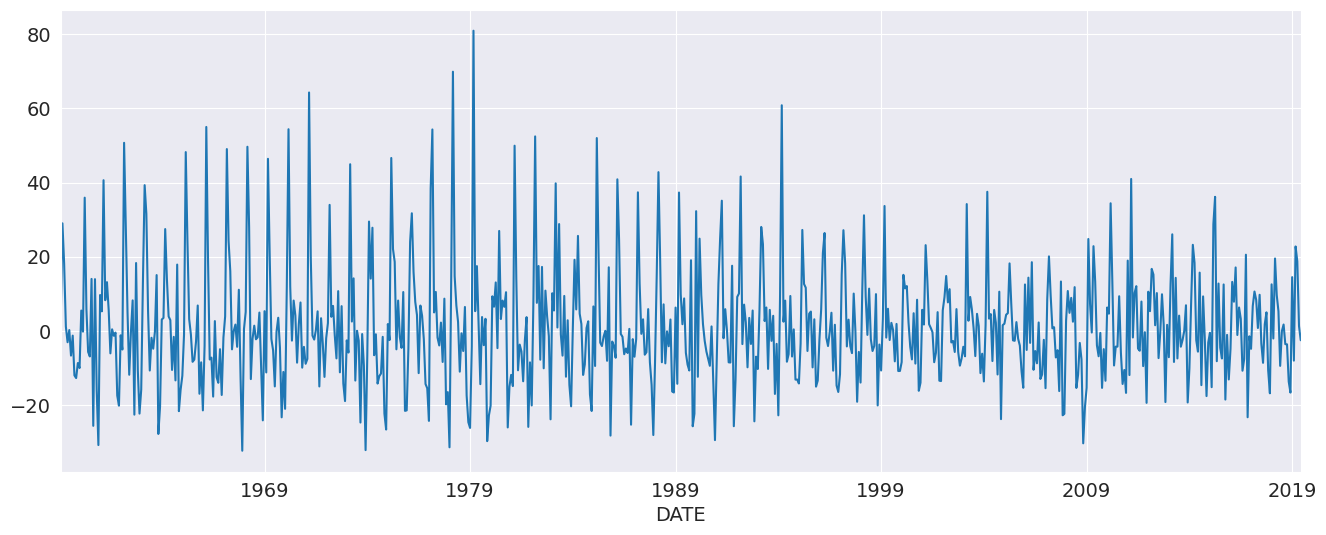We can start with an AR(3). While this is not a good model for this data, it demonstrates the basic use of the API.

:

mod = AutoReg(housing, 3)
res = mod.fit()
res.summary()

:

Dep. Variable: No. Observations: HOUSTNSA 725 AutoReg(3) -2993.442 Conditional MLE 15.289 Fri, 21 Feb 2020 5.468 13:53:22 5.500 05-01-1959 5.480 - 06-01-2019
coef std err z P>|z| [0.025 0.975] 1.1228 0.573 1.961 0.050 0.000 2.245 0.1910 0.036 5.235 0.000 0.120 0.263 0.0058 0.037 0.155 0.877 -0.067 0.079 -0.1939 0.036 -5.319 0.000 -0.265 -0.122
 Real Imaginary Modulus Frequency 0.9680 -1.3298j 1.6448 -0.1499 0.9680 +1.3298j 1.6448 0.1499 -1.9064 -0.0000j 1.9064 -0.5000

AutoReg supports the same covariance estimators as OLS. Below, we use cov_type="HC0", which is White’s covariance estimator. While the parameter estimates are the same, all of the quantities that depend on the standard error change.

:

res = mod.fit(cov_type="HC0")
res.summary()

:

Dep. Variable: No. Observations: HOUSTNSA 725 AutoReg(3) -2993.442 Conditional MLE 15.289 Fri, 21 Feb 2020 5.468 13:53:22 5.500 05-01-1959 5.480 - 06-01-2019
coef std err z P>|z| [0.025 0.975] 1.1228 0.601 1.869 0.062 -0.055 2.300 0.1910 0.035 5.499 0.000 0.123 0.259 0.0058 0.039 0.150 0.881 -0.070 0.081 -0.1939 0.036 -5.448 0.000 -0.264 -0.124
 Real Imaginary Modulus Frequency 0.9680 -1.3298j 1.6448 -0.1499 0.9680 +1.3298j 1.6448 0.1499 -1.9064 -0.0000j 1.9064 -0.5000
:

sel = ar_select_order(housing, 13)
sel.ar_lags
res = sel.model.fit()
res.summary()

:

Dep. Variable: No. Observations: HOUSTNSA 725 AutoReg(13) -2676.157 Conditional MLE 10.378 Fri, 21 Feb 2020 4.722 13:53:22 4.818 03-01-1960 4.759 - 06-01-2019
coef std err z P>|z| [0.025 0.975] 1.3615 0.458 2.970 0.003 0.463 2.260 -0.2900 0.036 -8.161 0.000 -0.360 -0.220 -0.0828 0.031 -2.652 0.008 -0.144 -0.022 -0.0654 0.031 -2.106 0.035 -0.126 -0.005 -0.1596 0.031 -5.166 0.000 -0.220 -0.099 -0.0434 0.031 -1.387 0.165 -0.105 0.018 -0.0884 0.031 -2.867 0.004 -0.149 -0.028 -0.0556 0.031 -1.797 0.072 -0.116 0.005 -0.1482 0.031 -4.803 0.000 -0.209 -0.088 -0.0926 0.031 -2.960 0.003 -0.154 -0.031 -0.1133 0.031 -3.665 0.000 -0.174 -0.053 0.1151 0.031 3.699 0.000 0.054 0.176 0.5352 0.031 17.133 0.000 0.474 0.596 0.3178 0.036 8.937 0.000 0.248 0.388
 Real Imaginary Modulus Frequency 1.0913 -0.0000j 1.0913 -0.0000 0.8743 -0.5018j 1.0080 -0.0829 0.8743 +0.5018j 1.0080 0.0829 0.5041 -0.8765j 1.0111 -0.1669 0.5041 +0.8765j 1.0111 0.1669 0.0056 -1.0530j 1.0530 -0.2491 0.0056 +1.0530j 1.0530 0.2491 -0.5263 -0.9335j 1.0716 -0.3317 -0.5263 +0.9335j 1.0716 0.3317 -0.9525 -0.5880j 1.1194 -0.4120 -0.9525 +0.5880j 1.1194 0.4120 -1.2928 -0.2608j 1.3189 -0.4683 -1.2928 +0.2608j 1.3189 0.4683

plot_predict visualizes forecasts. Here we produce a large number of forecasts which show the string seasonality captured by the model.

:

fig = res.plot_predict(720, 840)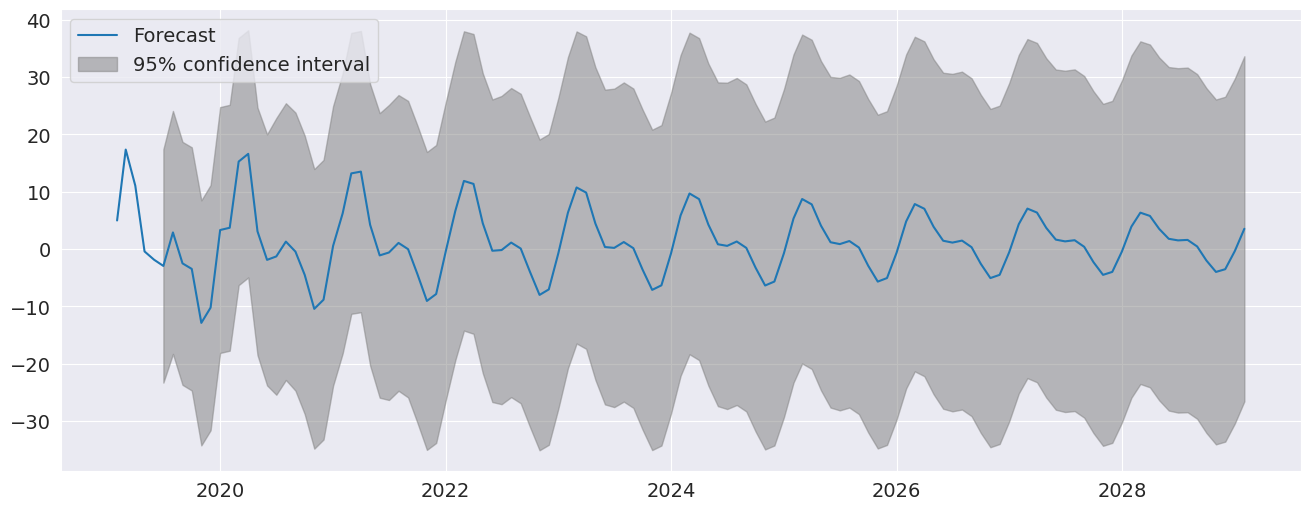plot_diagnositcs indicates that the model captures the key features in the data.

:

fig = plt.figure(figsize=(16,9))
fig = res.plot_diagnostics(fig=fig, lags=30)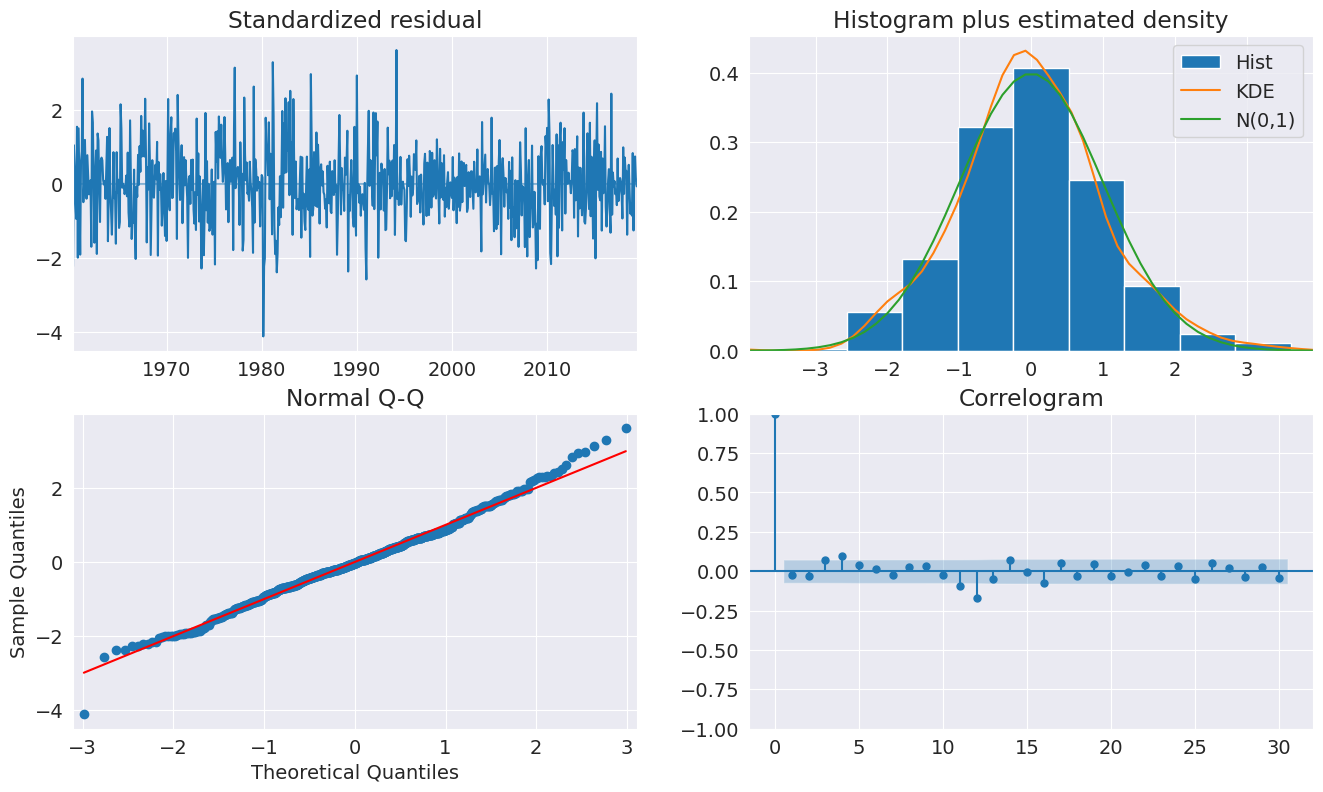## Seasonal Dummies¶

AutoReg supports seasonal dummies which are an alternative way to model seasonality. Including the dummies shortens the dynamics to only an AR(2).

:

sel = ar_select_order(housing, 13, seasonal=True)
sel.ar_lags
res = sel.model.fit()
res.summary()

:

Dep. Variable: No. Observations: HOUSTNSA 725 Seas. AutoReg(2) -2652.556 Conditional MLE 9.487 Fri, 21 Feb 2020 4.541 13:53:24 4.636 04-01-1959 4.578 - 06-01-2019
coef std err z P>|z| [0.025 0.975] 1.2726 1.373 0.927 0.354 -1.418 3.963 32.6477 1.824 17.901 0.000 29.073 36.222 23.0685 2.435 9.472 0.000 18.295 27.842 10.7267 2.693 3.983 0.000 5.449 16.005 1.6792 2.100 0.799 0.424 -2.437 5.796 -4.4229 1.896 -2.333 0.020 -8.138 -0.707 -4.2113 1.824 -2.309 0.021 -7.786 -0.636 -6.4124 1.791 -3.581 0.000 -9.922 -2.902 0.1095 1.800 0.061 0.952 -3.419 3.638 -16.7511 1.814 -9.234 0.000 -20.307 -13.196 -20.7023 1.862 -11.117 0.000 -24.352 -17.053 -11.9554 1.778 -6.724 0.000 -15.440 -8.470 -0.2953 0.037 -7.994 0.000 -0.368 -0.223 -0.1148 0.037 -3.107 0.002 -0.187 -0.042
 Real Imaginary Modulus Frequency -1.2862 -2.6564j 2.9514 -0.3218 -1.2862 +2.6564j 2.9514 0.3218

The seasonal dummies are obvious in the forecasts which has a non-trivial seasonal component in all periods 10 years in to the future.

:

fig = res.plot_predict(720, 840)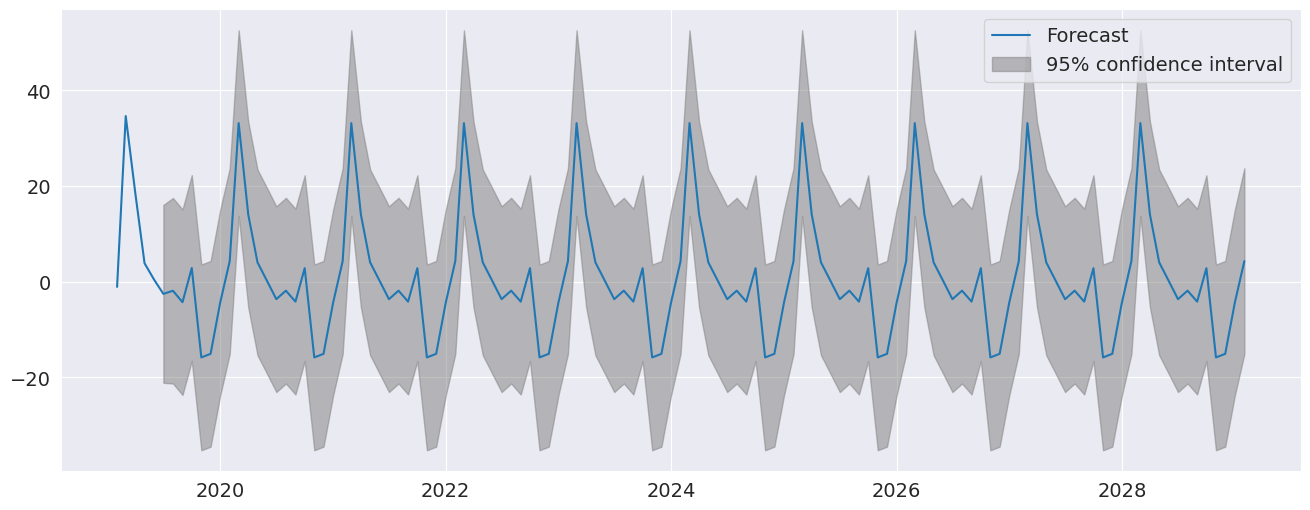:

fig = plt.figure(figsize=(16,9))
fig = res.plot_diagnostics(lags=30, fig=fig)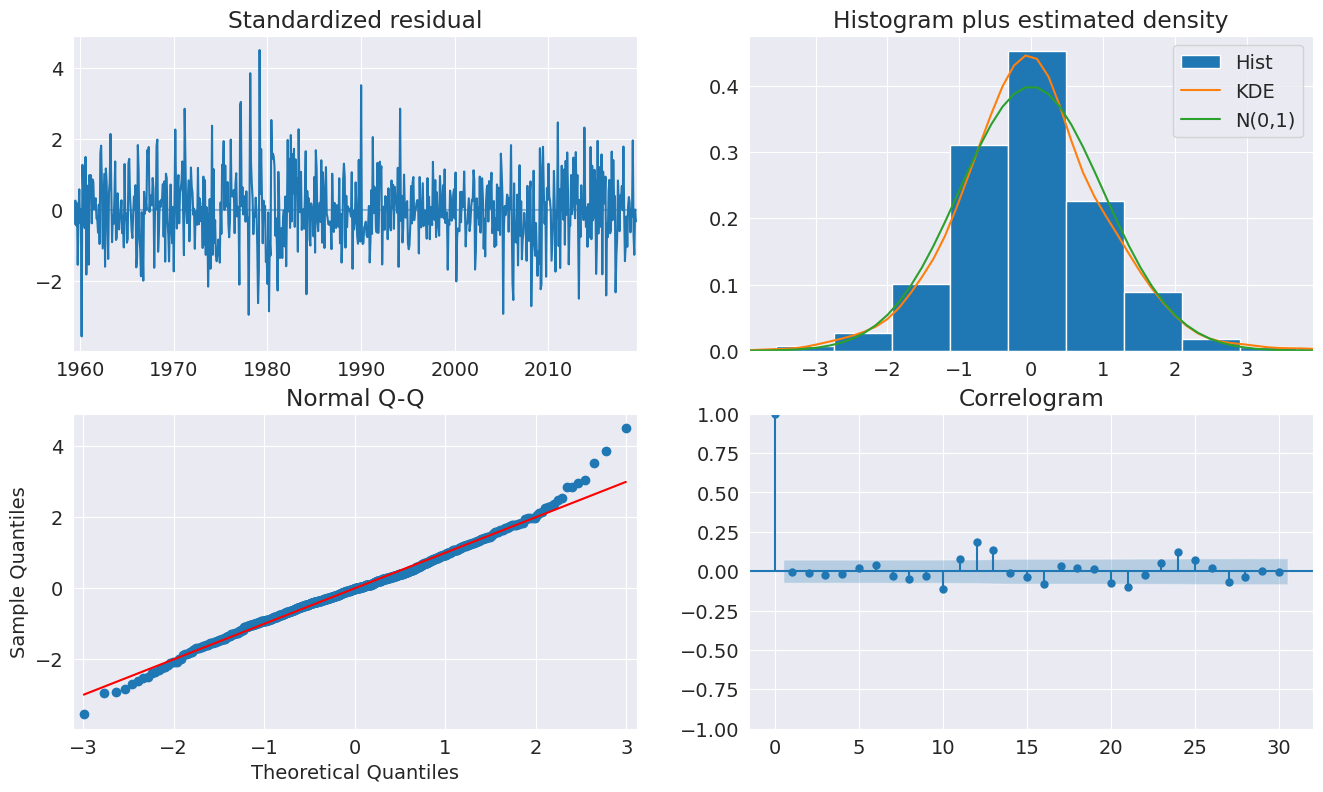## Seasonal Dynamics¶

While AutoReg does not directly support Seasonal components since it uses OLS to estimate parameters, it is possible to capture seasonal dynamics using an over-parametrized Seasonal AR that does not impose the restrictions in the Seasonal AR.

:

yoy_housing = data.HOUSTNSA.pct_change(12).resample("MS").last().dropna()
_, ax = plt.subplots()
ax = yoy_housing.plot(ax=ax)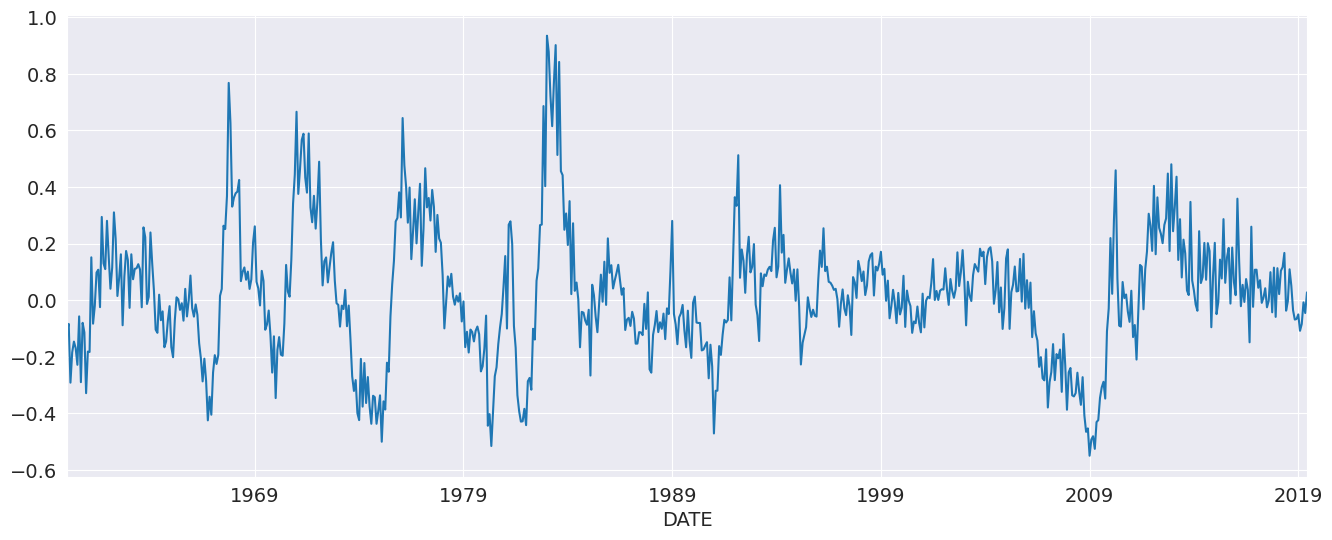We start by selecting a model using the simple method that only chooses the maximum lag. All lower lags are automatically included. The maximum lag to check is set to 13 since this allows the model to next a Seasonal AR that has both a short-run AR(1) component and a Seasonal AR(1) component, so that

\begin{align}\begin{aligned}(1-\phi_s L^{12})(1-\phi_1 L)y_t = \epsilon_t\\which becomes\end{aligned}\end{align}
$y_t = \phi_1 y_{t-1} +\phi_s Y_{t-12} - \phi_1\phi_s Y_{t-13} + \epsilon_t$

when expanded. AutoReg does not enforce the structure, but can estimate the nesting model

$y_t = \phi_1 y_{t-1} +\phi_{12} Y_{t-12} - \phi_{13} Y_{t-13} + \epsilon_t.$

We see that all 13 lags are selected.

:

sel = ar_select_order(yoy_housing, 13)
sel.ar_lags

:

array([ 1,  2,  3,  4,  5,  6,  7,  8,  9, 10, 11, 12, 13])


It seems unlikely that all 13 lags are required. We can set glob=True to search all $$2^{13}$$ models that include up to 13 lags.

Here we see that the first three are selected, as is the 7th, and finally the 12th and 13th are selected. This is superficially similar to the structure described above.

After fitting the model, we take a look at the diagnostic plots that indicate that this specification appears to be adequate to capture the dynamics in the data.

:

sel = ar_select_order(yoy_housing, 13, glob=True)
sel.ar_lags
res = sel.model.fit()
res.summary()

:

Dep. Variable: No. Observations: HOUSTNSA 714 Restr. AutoReg(13) 589.177 Conditional MLE 0.104 Fri, 21 Feb 2020 -4.496 13:53:33 -4.444 02-01-1961 -4.476 - 06-01-2019
coef std err z P>|z| [0.025 0.975] 0.0035 0.004 0.875 0.382 -0.004 0.011 0.5640 0.035 16.167 0.000 0.496 0.632 0.2347 0.038 6.238 0.000 0.161 0.308 0.2051 0.037 5.560 0.000 0.133 0.277 -0.0903 0.030 -2.976 0.003 -0.150 -0.031 -0.3791 0.034 -11.075 0.000 -0.446 -0.312 0.3354 0.033 10.254 0.000 0.271 0.400
 Real Imaginary Modulus Frequency -1.0309 -0.2682j 1.0652 -0.4595 -1.0309 +0.2682j 1.0652 0.4595 -0.7454 -0.7417j 1.0515 -0.3754 -0.7454 +0.7417j 1.0515 0.3754 -0.3172 -1.0221j 1.0702 -0.2979 -0.3172 +1.0221j 1.0702 0.2979 0.2419 -1.0573j 1.0846 -0.2142 0.2419 +1.0573j 1.0846 0.2142 0.7840 -0.8303j 1.1420 -0.1296 0.7840 +0.8303j 1.1420 0.1296 1.0730 -0.2386j 1.0992 -0.0348 1.0730 +0.2386j 1.0992 0.0348 1.1193 -0.0000j 1.1193 -0.0000
:

fig = plt.figure(figsize=(16,9))
fig = res.plot_diagnostics(fig=fig, lags=30)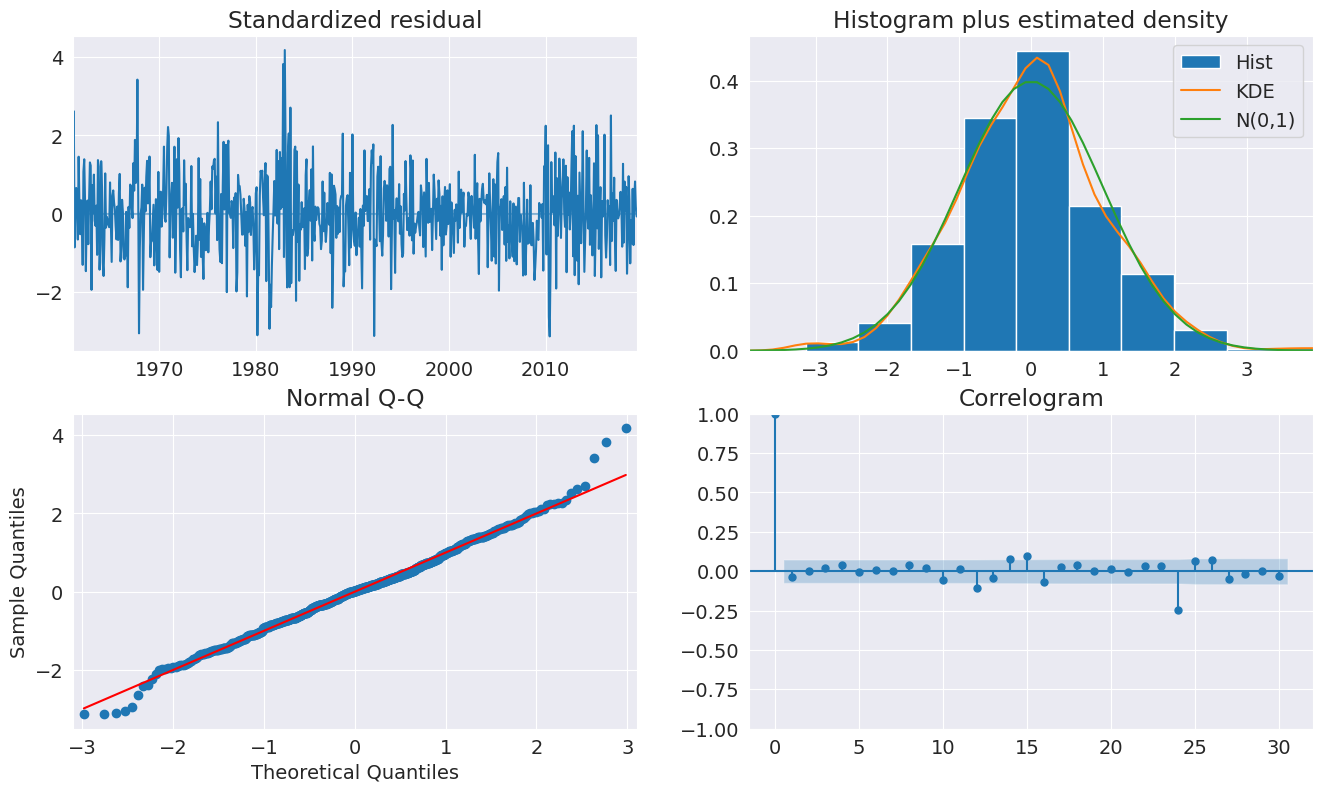We can also include seasonal dummies. These are all insignificant since the model is using year-over-year changes.

:

sel = ar_select_order(yoy_housing, 13, glob=True, seasonal=True)
sel.ar_lags
res = sel.model.fit()
res.summary()

:

Dep. Variable: No. Observations: HOUSTNSA 714 Restr. Seas. AutoReg(13) 590.875 Conditional MLE 0.104 Fri, 21 Feb 2020 -4.469 13:53:44 -4.346 02-01-1961 -4.422 - 06-01-2019
coef std err z P>|z| [0.025 0.975] 0.0167 0.014 1.215 0.224 -0.010 0.044 -0.0179 0.019 -0.931 0.352 -0.056 0.020 -0.0121 0.019 -0.630 0.528 -0.050 0.026 -0.0210 0.019 -1.089 0.276 -0.059 0.017 -0.0223 0.019 -1.157 0.247 -0.060 0.015 -0.0224 0.019 -1.160 0.246 -0.060 0.015 -0.0212 0.019 -1.096 0.273 -0.059 0.017 -0.0101 0.019 -0.520 0.603 -0.048 0.028 -0.0095 0.019 -0.491 0.623 -0.047 0.028 -0.0049 0.019 -0.252 0.801 -0.043 0.033 -0.0084 0.019 -0.435 0.664 -0.046 0.030 -0.0077 0.019 -0.400 0.689 -0.046 0.030 0.5630 0.035 16.160 0.000 0.495 0.631 0.2347 0.038 6.248 0.000 0.161 0.308 0.2075 0.037 5.634 0.000 0.135 0.280 -0.0916 0.030 -3.013 0.003 -0.151 -0.032 -0.3810 0.034 -11.149 0.000 -0.448 -0.314 0.3373 0.033 10.327 0.000 0.273 0.401
 Real Imaginary Modulus Frequency -1.0305 -0.2681j 1.0648 -0.4595 -1.0305 +0.2681j 1.0648 0.4595 -0.7447 -0.7414j 1.0509 -0.3754 -0.7447 +0.7414j 1.0509 0.3754 -0.3172 -1.0215j 1.0696 -0.2979 -0.3172 +1.0215j 1.0696 0.2979 0.2416 -1.0568j 1.0841 -0.2142 0.2416 +1.0568j 1.0841 0.2142 0.7837 -0.8304j 1.1418 -0.1296 0.7837 +0.8304j 1.1418 0.1296 1.0724 -0.2383j 1.0986 -0.0348 1.0724 +0.2383j 1.0986 0.0348 1.1192 -0.0000j 1.1192 -0.0000

## Industrial Production¶

We will use the industrial production index data to examine forecasting.

:

data = pdr.get_data_fred('INDPRO', '1959-01-01', '2019-06-01')
ind_prod = data.INDPRO.pct_change(12).dropna().asfreq('MS')
_, ax = plt.subplots(figsize=(16,9))
ind_prod.plot(ax=ax)

:

<matplotlib.axes._subplots.AxesSubplot at 0x7fec8d4cd5d0>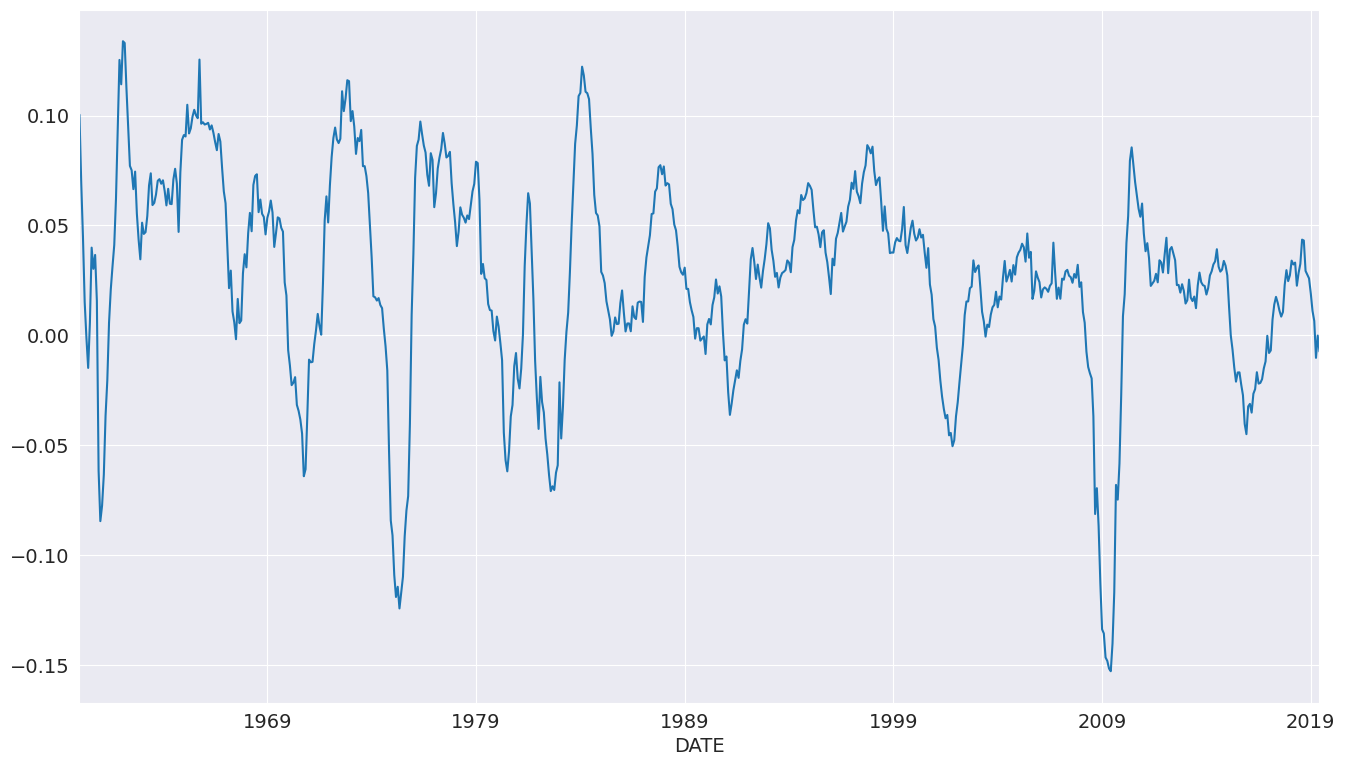We will start by selecting a model using up to 12 lags. An AR(13) minimizes the BIC criteria even though many coefficients are insignificant.

:

sel = ar_select_order(ind_prod, 13, 'bic')
res = sel.model.fit()
res.summary()

:

Dep. Variable: No. Observations: INDPRO 714 AutoReg(13) 2318.848 Conditional MLE 0.009 Fri, 21 Feb 2020 -9.411 13:53:45 -9.313 02-01-1961 -9.373 - 06-01-2019
coef std err z P>|z| [0.025 0.975] 0.0012 0.000 2.896 0.004 0.000 0.002 1.1504 0.035 32.940 0.000 1.082 1.219 -0.0747 0.053 -1.407 0.159 -0.179 0.029 0.0043 0.053 0.081 0.935 -0.099 0.107 0.0027 0.053 0.052 0.959 -0.100 0.106 -0.1383 0.052 -2.642 0.008 -0.241 -0.036 0.0085 0.052 0.163 0.871 -0.094 0.111 0.0375 0.052 0.720 0.471 -0.065 0.139 -0.0235 0.052 -0.453 0.651 -0.125 0.078 0.0945 0.052 1.824 0.068 -0.007 0.196 -0.0844 0.052 -1.627 0.104 -0.186 0.017 0.0024 0.052 0.047 0.962 -0.099 0.104 -0.3809 0.052 -7.367 0.000 -0.482 -0.280 0.3589 0.033 10.916 0.000 0.294 0.423
 Real Imaginary Modulus Frequency -1.0411 -0.2889j 1.0804 -0.4569 -1.0411 +0.2889j 1.0804 0.4569 -0.7774 -0.8064j 1.1201 -0.3721 -0.7774 +0.8064j 1.1201 0.3721 -0.2760 -1.0530j 1.0886 -0.2908 -0.2760 +1.0530j 1.0886 0.2908 0.2719 -1.0515j 1.0861 -0.2097 0.2719 +1.0515j 1.0861 0.2097 0.8019 -0.7297j 1.0842 -0.1175 0.8019 +0.7297j 1.0842 0.1175 1.0224 -0.2214j 1.0461 -0.0339 1.0224 +0.2214j 1.0461 0.0339 1.0578 -0.0000j 1.0578 -0.0000

We can also use a global search which allows longer lags to enter if needed without requiring the shorter lags. Here we see many lags dropped. The model indicates there may be some seasonality in the data.

:

sel = ar_select_order(ind_prod, 13, 'bic', glob=True)
sel.ar_lags
res_glob = sel.model.fit()
res_glob.summary()

:

Dep. Variable: No. Observations: INDPRO 714 Restr. AutoReg(13) 2313.420 Conditional MLE 0.009 Fri, 21 Feb 2020 -9.421 13:53:53 -9.382 02-01-1961 -9.406 - 06-01-2019
coef std err z P>|z| [0.025 0.975] 0.0013 0.000 2.986 0.003 0.000 0.002 1.0882 0.013 80.869 0.000 1.062 1.115 -0.1057 0.016 -6.649 0.000 -0.137 -0.075 -0.3852 0.034 -11.404 0.000 -0.451 -0.319 0.3587 0.032 11.315 0.000 0.297 0.421
 Real Imaginary Modulus Frequency -1.0518 -0.2762j 1.0875 -0.4591 -1.0518 +0.2762j 1.0875 0.4591 -0.7718 -0.7796j 1.0970 -0.3742 -0.7718 +0.7796j 1.0970 0.3742 -0.2714 -1.0479j 1.0825 -0.2903 -0.2714 +1.0479j 1.0825 0.2903 0.2820 -1.0573j 1.0943 -0.2085 0.2820 +1.0573j 1.0943 0.2085 0.8021 -0.7558j 1.1021 -0.1203 0.8021 +0.7558j 1.1021 0.1203 1.0189 -0.2198j 1.0423 -0.0338 1.0189 +0.2198j 1.0423 0.0338 1.0578 -0.0000j 1.0578 -0.0000

plot_predict can be used to produce forecast plots along with confidence intervals. Here we produce forecasts starting at the last observation and continuing for 18 months.

:

ind_prod.shape

:

(714,)

:

fig = res_glob.plot_predict(start=714, end=732)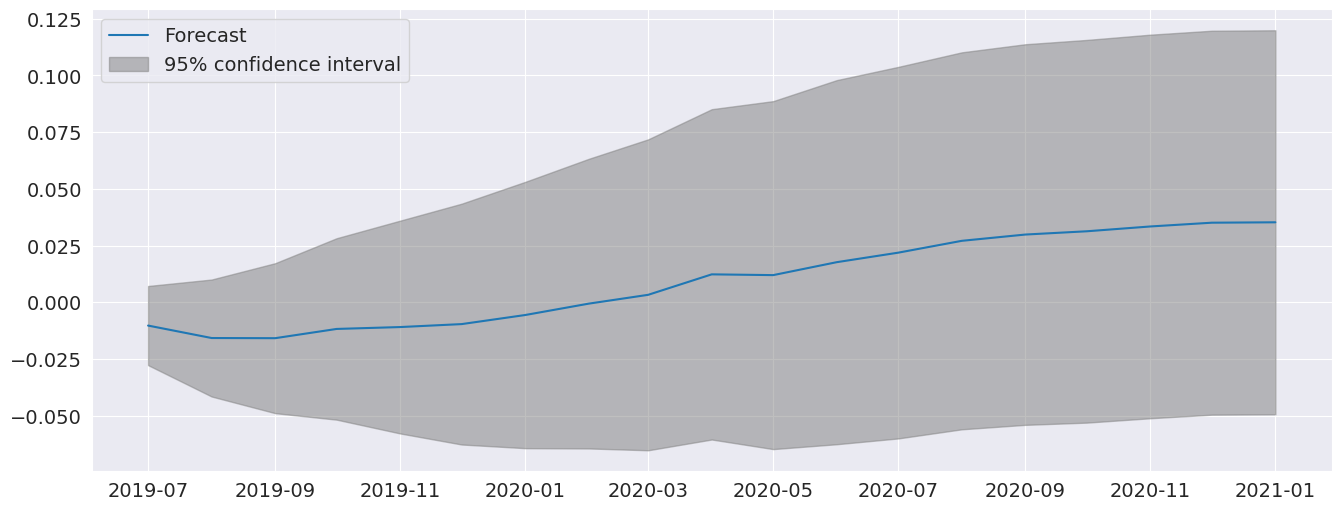The forecasts from the full model and the restricted model are very similar. I also include an AR(5) which has very different dynamics

:

res_ar5 = AutoReg(ind_prod, 5).fit()
predictions = pd.DataFrame({"AR(5)": res_ar5.predict(start=714, end=726),
"AR(13)": res.predict(start=714, end=726),
"Restr. AR(13)": res_glob.predict(start=714, end=726)})
_, ax = plt.subplots()
ax = predictions.plot(ax=ax)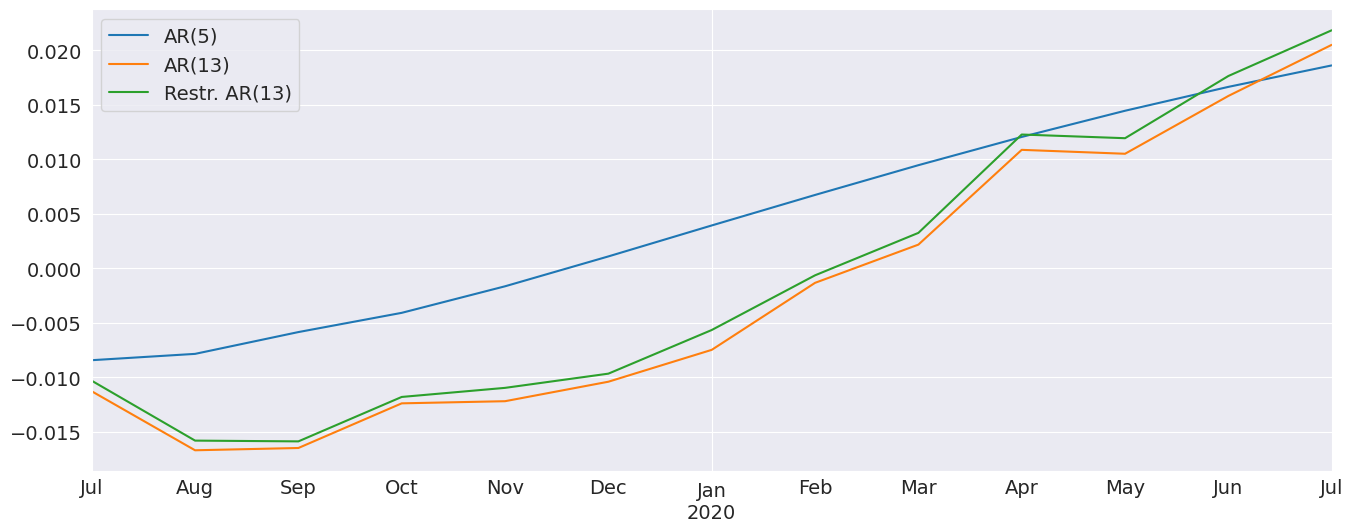The diagnostics indicate the model captures most of the the dynamics in the data. The ACF shows a patters at the seasonal frequency and so a more complete seasonal model (SARIMAX) may be needed.

:

fig = plt.figure(figsize=(16,9))
fig = res_glob.plot_diagnostics(fig=fig, lags=30)# Forecasting¶

Forecasts are produced using the predict method from a results instance. The default produces static forecasts which are one-step forecasts. Producing multi-step forecasts requires using dynamic=True.

In this next cell, we produce 12-step-heard forecasts for the final 24 periods in the sample. This requires a loop.

Note: These are technically in-sample since the data we are forecasting was used to estimate parameters. Producing OOS forecasts requires two models. The first must exclude the OOS period. The second uses the predict method from the full-sample model with the parameters from the shorter sample model that excluded the OOS period.

:

import numpy as np
start = ind_prod.index[-24]
forecast_index = pd.date_range(start, freq=ind_prod.index.freq, periods=36)
cols = ['-'.join(str(val) for val in (idx.year, idx.month)) for idx in forecast_index]
forecasts = pd.DataFrame(index=forecast_index,columns=cols)
for i in range(1, 24):
fcast = res_glob.predict(start=forecast_index[i], end=forecast_index[i+12], dynamic=True)
forecasts.loc[fcast.index, cols[i]] = fcast
_, ax = plt.subplots(figsize=(16, 10))
ind_prod.iloc[-24:].plot(ax=ax, color="black", linestyle="--")
ax = forecasts.plot(ax=ax)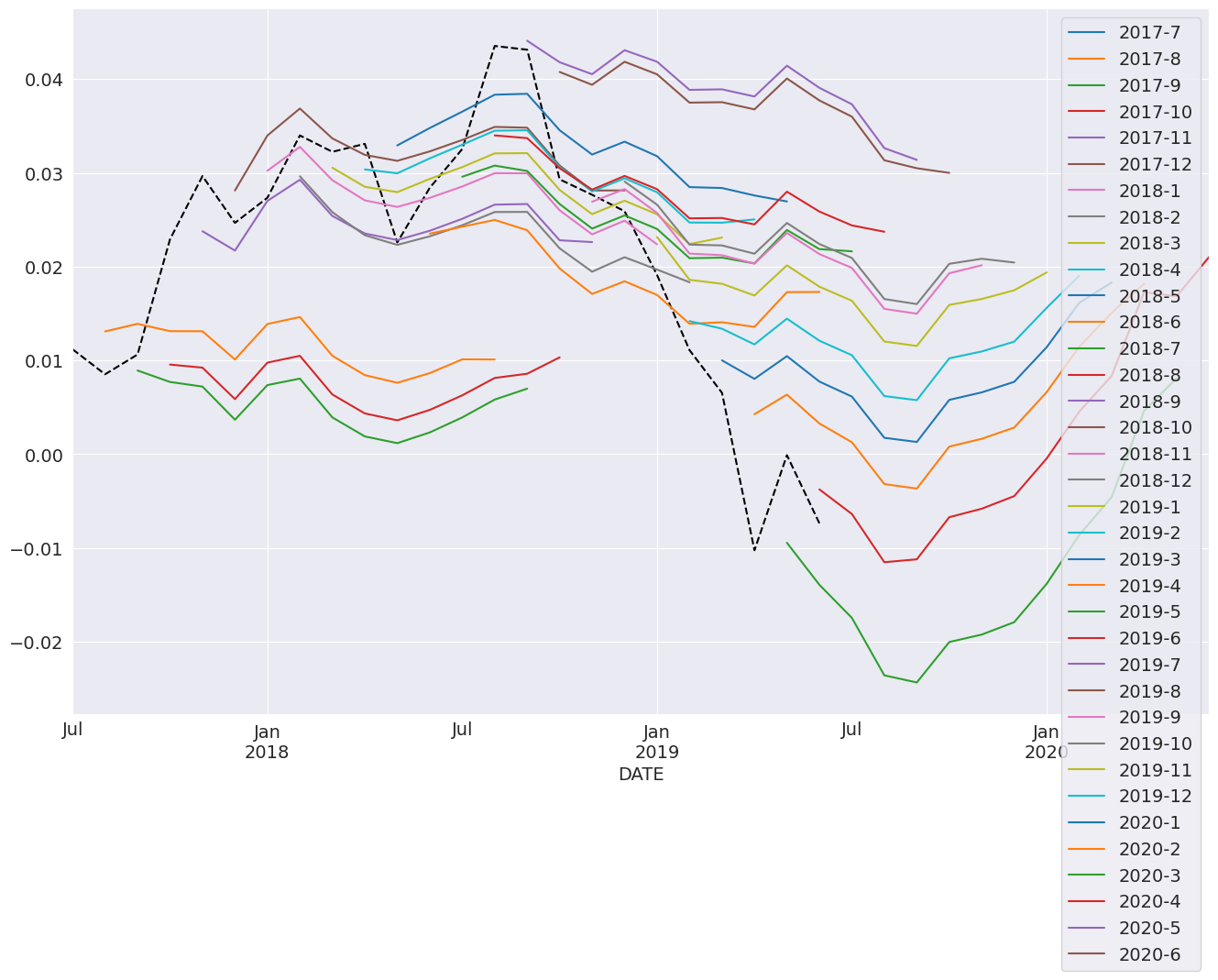## Comparing to SARIMAX¶

SARIMAX is an implementation of a Seasonal Autoregressive Integrated Moving Average with eXogenous regressors model. It supports:

• Specification of seasonal and nonseasonal AR and MA components

• Inclusion of Exogenous variables

• Full maximum-likelihood estimation using the Kalman Filter

This model is more feature rich than AutoReg. Unlike SARIMAX, AutoReg estimates parameters using OLS. This is faster and the problem is globally convex, and so there are no issues with local minima. The closed-form estimator and its performance are the key advantages of AutoReg over SARIMAX when comparing AR(P) models. AutoReg also support seasonal dummies, which can be used with SARIMAX if the user includes them as exogenous regressors.

:

from statsmodels.tsa.api import SARIMAX

sarimax_mod = SARIMAX(ind_prod, order=((1,5,12,13),0, 0), trend='c')
sarimax_res = sarimax_mod.fit()
sarimax_res.summary()

:

Dep. Variable: No. Observations: INDPRO 714 SARIMAX([1, 5, 12, 13], 0, 0) 2302.146 Fri, 21 Feb 2020 -4592.293 13:54:00 -4564.867 01-01-1960 -4581.701 - 06-01-2019 opg
coef std err z P>|z| [0.025 0.975] 0.0011 0.000 2.627 0.009 0.000 0.002 1.0798 0.010 106.388 0.000 1.060 1.100 -0.0849 0.011 -7.467 0.000 -0.107 -0.063 -0.4392 0.026 -16.935 0.000 -0.490 -0.388 0.4032 0.025 15.831 0.000 0.353 0.453 9.179e-05 3.13e-06 29.366 0.000 8.57e-05 9.79e-05
 Ljung-Box (Q): Jarque-Bera (JB): 177.22 928.85 0 0 0.38 -0.63 0 8.44

Warnings:
 Covariance matrix calculated using the outer product of gradients (complex-step).
:

sarimax_params = sarimax_res.params.iloc[:-1].copy()
sarimax_params.index = res_glob.params.index
params = pd.concat([res_glob.params, sarimax_params], axis=1, sort=False)
params.columns = ["AutoReg", "SARIMAX"]
params

:

AutoReg SARIMAX
intercept 0.001292 0.001140
INDPRO.L1 1.088215 1.079829
INDPRO.L5 -0.105668 -0.084904
INDPRO.L12 -0.385171 -0.439175
INDPRO.L13 0.358738 0.403241